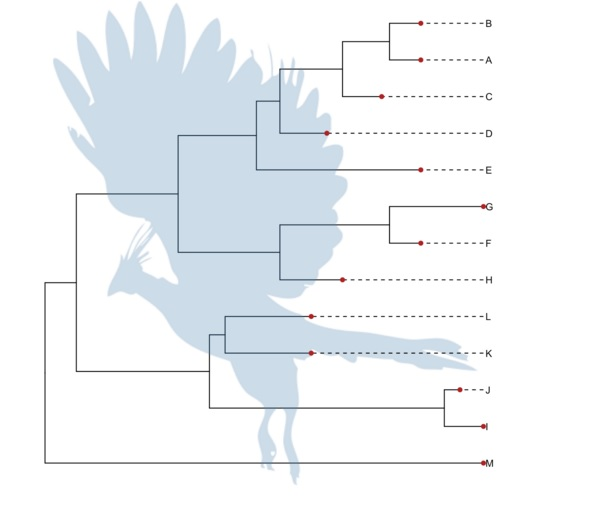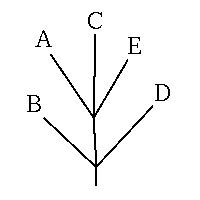(B,(A,C,E),D);


(B:6.0,(A:5.0,C:3.0,E:4.0):5.0,D:11.0);


(B:6.0,(A:5.0,C:3.0,E:4.0)Ancestor1:5.0,D:11.0);


ggtree的开发源自于我需要在树上做注释，发现并没有软件可以很容易地实现，通常情况下我们把统计信息加到节点的label上来展示，比如CodeML的dN/dS分析，输出文件里就给用户准备了newick树文本，把dN/dS ( $$\omega$$ ) 加于节点label之上:

codeml_file <-system.file("extdata/PAML_Codeml/mlc", package="ggtree")
tree_text

#  "w ratios as labels for TreeView:"
#  "(K #0.0224 , N #0.0095 , (D #0.0385 , (L #0.0001 , (J #0.0457 , (G #0.1621 , ((C #0.0461 , (E #0.0641 , O #0.0538 ) #0.0001 ) #0.0395 , (H #0.1028 , (I #0.0001 , (B #0.0001 , (A #0.0646 , (F #0.2980 , M #0.0738 ) #0.0453 ) #0.0863 ) #1.5591 ) #0.0001 ) #0.0001 ) #0.0549 ) #0.0419 ) #0.0001 ) #0.0964 ) #0.0129 );"


ggtree(read.tree(file))


ggtree的设计

# 扩展ggplot2

if (show.tip.label) {
p <- p + geom_text(data = df.tip, aes(x = x, y = y, label = label),
hjust = 0, size = tip.label.size)
}


ggtree是真正扩展ggplot2，支持图形语法的包。我们首先扩展ggplot支持tree object做为输入，并实现geom_tree图层来画线条。

library(ggplot2)
library(ggtree)
set.seed(2015-11-26)
tree <-rtree(30)
ggplot(tree, aes(x, y)) + geom_tree()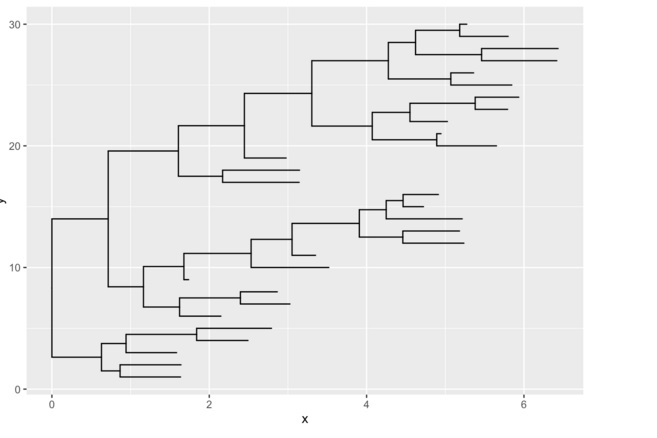ggtree函数是 ggplot() + geom_tree() + xlab(NA) + ylab(NA) + theme_tree() 的简单组合。

ggtree(tree)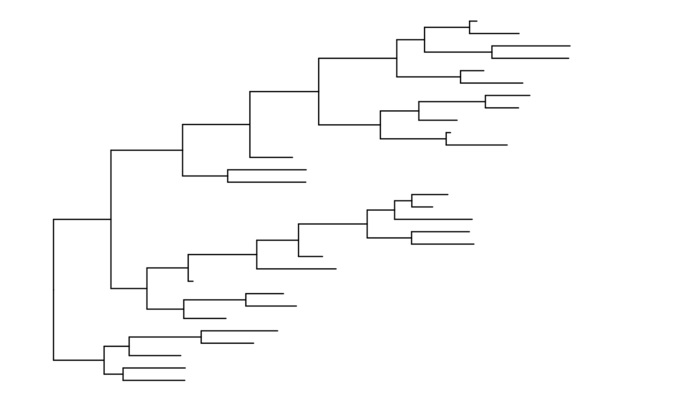ggtree(tree) + geom_tiplab() + geom_point(color='firebrick')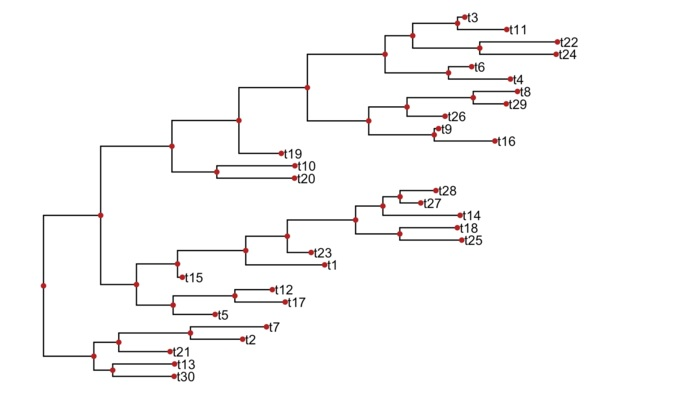树的操作与注释

nwk <- system.file("extdata", "sample.nwk", package="ggtree")
p <- ggtree(tree)
cp <- ggtree(tree) %>% collapse(node=21) + ggtitle('collapse')
ep <- cp + expand(node=21) + ggtitle('expand')
hp <- p + hilight(node=21) + ggtitle('hilight')
rp <- hp + rotate(node=21) + ggtitle('rotate')
library(gridExtra)
grid.arrange(cp, ep, hp, rp, ncol=2)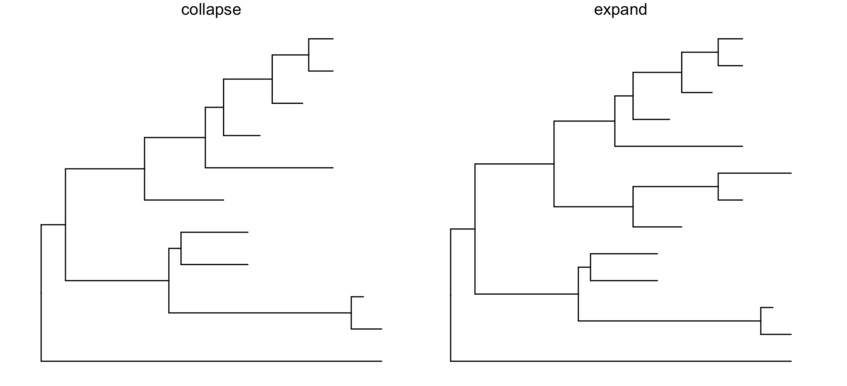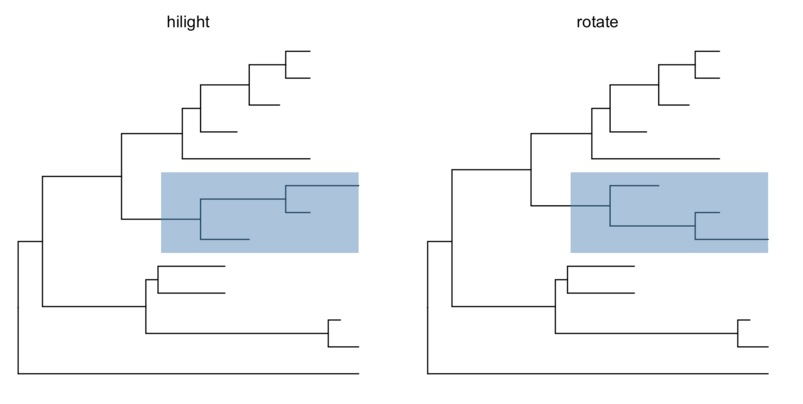## 支持多种文件格式

ggtree支持的文件格式包括Newick, Nexus, NHX和jplace。

nhxfile = system.file("extdata", "ADH.nhx", package="ggtree")
ggtree(nhx, ladderize=F) + geom_tiplab() + geom_point(aes(color=S), size=8, alpha=.3) +
theme(legend.position="right") +
geom_text(aes(label=branch.length, x=branch), vjust=-.5) +
xlim(NA, 0.3)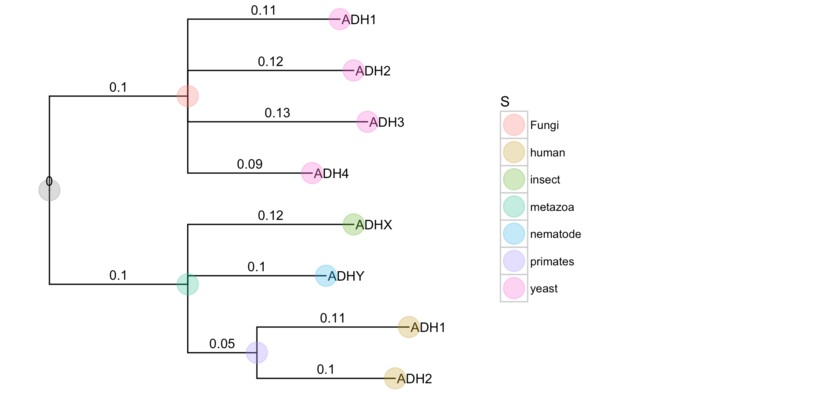支持解析多种软件的输出文件

ggtree支持ape, phangorn, r8s, RAxML, PAML, HYPHY, EPA, pplacer和BEAST的输出。相应的统计分析结果可以应用于树的注释。可以说ggtree把这些软件分析的结果带给了R用户，通过ggtree的解析， 这些进化分析结果可以进一点在R里进行处理和统计分析，并不单单是在ggtree中展示而已。

### RAxML bootstrap分析

raxml_file <-system.file("extdata/RAxML", "RAxML_bipartitionsBranchLabels.H3", package="ggtree")
ggtree(raxml) + geom_text(aes(label=bootstrap, color=bootstrap)) +
theme(legend.position='right')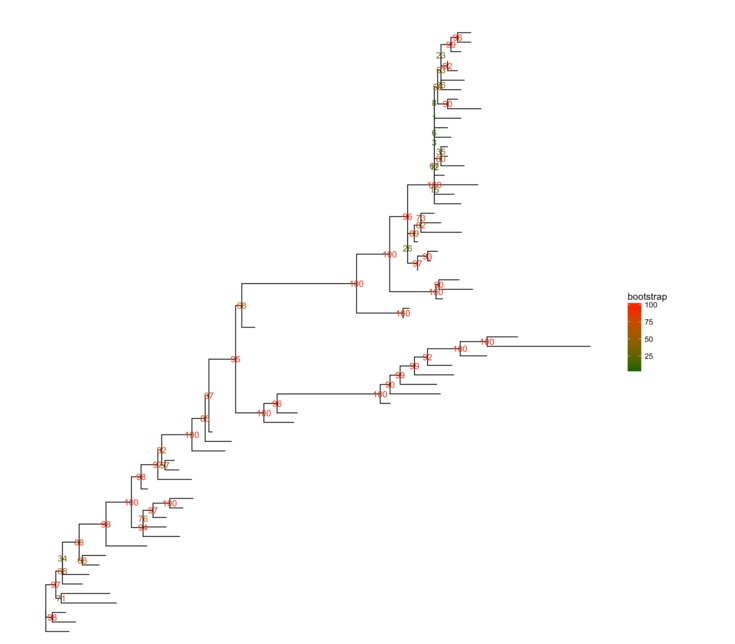multiPhylo也是支持的，所以100颗bootstrap树可以同时用一行代码展示出来。

btree_file <-system.file("extdata/RAxML", "RAxML_bootstrap.H3", package="ggtree")
ggtree(btree) + facet_wrap(~.id, ncol=10)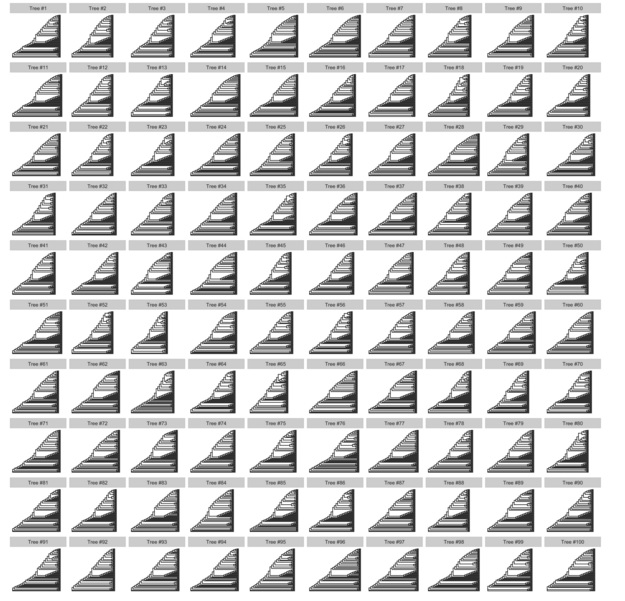### PAML

rstfile <-system.file("extdata/PAML_Baseml", "rst", package="ggtree")
p <-ggtree(rst) + geom_text(aes(label=marginal_AA_subs, x=branch), vjust=-.5)
print(p)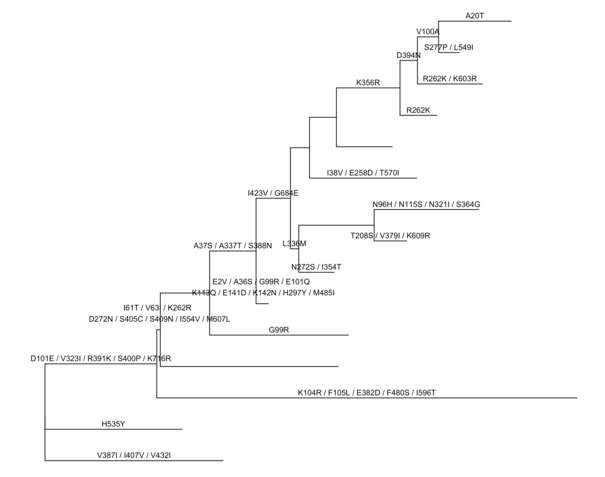rstfile <-system.file("extdata/PAML_Codeml", "rst", package="ggtree")
p %<% crst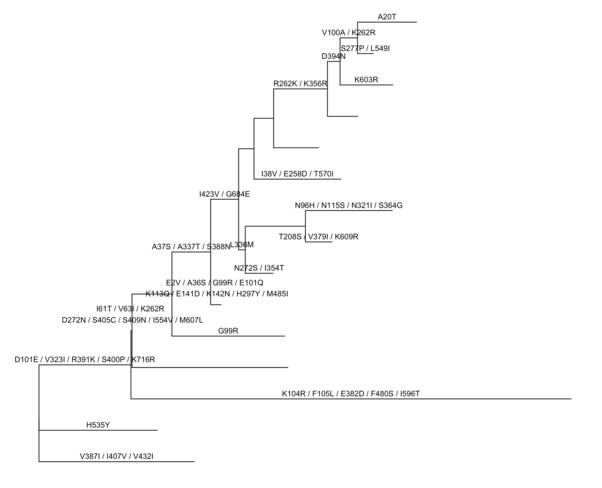CodeML的dN/dS分析，我们可以直接把数据拿来给树上色。同样道理分类数据也可以拿来上色。

mlc_file <-system.file("examples/mlc", package="ggtree")
ggtree(mlc, aes(color=dN_vs_dS)) +
scale_color_continuous(limits=c(0, 1.5), high='red', low='green', oob=scales::squish, name='dN/dS') +
theme(legend.position='right')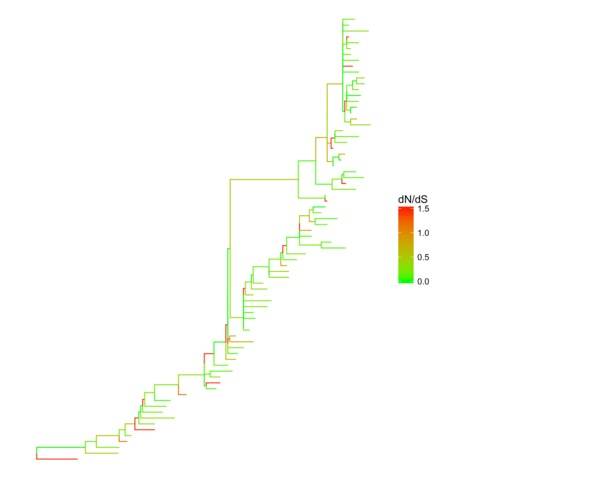使用用户定义数据

nwk <-system.file("extdata", "sample.nwk", package="ggtree")
p <-ggtree(tree)
dd <-data.frame(taxa = LETTERS[1:13],
place = c(rep("GZ", 5), rep("HK", 3), rep("CZ", 4), NA),
value = round(abs(rnorm(13, mean=70, sd=10)), digits=1))
## you don't need to order the data
## data was reshuffled just for demonstration
dd <-dd[sample(1:13, 13), ]
row.names(dd) <- NULL
print(dd)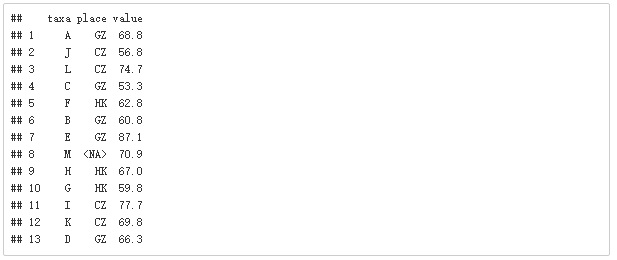p <- p %<+% dd + geom_text(aes(color=place, label=label), hjust=-0.5) +
geom_tippoint(aes(size=value, shape=place, color=place), alpha=0.25)
p+theme(legend.position="right")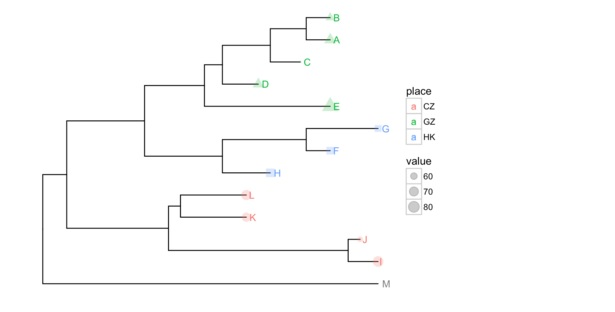ggtree还支持用户把自己的数据和树保存为jplace格式。

ggtree允许把不同软件的分析结果整合在一起，同时在树上展示或者比较结果。在我们提交的论文中，使用了整合BEAST和CodeML的例子，在树上展示dN/dS、时间轴、氨基酸替换、clade support values、物种和基因型 (genotype）等多维信息，6种不同的信息同时展示在一颗进化树上，这是个复杂的例子，我们在附件1中展示了可重复的代码。如果有兴趣，可以留意一下我们的文章。 🙂

## 其它好玩的功能

pp <-ggtree(tree) %<+% phylopic("79ad5f09-cf21-4c89-8e7d-0c82a00ce728", color="steelblue", alpha = .3)
pp + geom_tiplab(align=T, linetype='dashed', linesize=.5) + geom_tippoint(color='firebrick', size=2)